# Using R-DOE and ALTA to Estimate B10 Life

This example appears in the Experiment Design and Analysis Reference book.

Consider a product whose reliability is thought to be affected by eight potential factors:$A\,\!$ (temperature),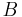$B\,\!$ (humidity),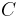$C\,\!$ (load),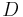$D\,\!$ (fan-speed),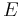$E\,\!$ (voltage),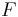$F\,\!$ (material),$G\,\!$ (vibration) and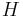$H\,\!$ (current). Assuming that all interaction effects are absent, a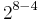$2^{8-4}\,\!$ design is used to investigate the eight factors at two levels. The generators used to obtain the design are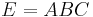$E=ABC\,\!$,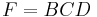$F=BCD\,\!$,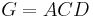$G=ACD\,\!$ and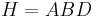$H=ABD\,\!$. The design and the corresponding life data obtained are shown next.

Readings for the experiment are taken every 20 hours and the test is terminated at 200 hours. The life of the product is assumed to follow the Weibull distribution.

The results from Weibull++ for this experiment are shown next.

The results show that only factors$A\,\!$ and$D\,\!$ are active at a significance level of 0.1.

Assume that, in terms of the actual units, the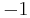$-1\,\!$ level of factor$A\,\!$ corresponds to a temperature of 333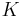$K\,\!$ and the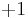$+1\,\!$ level corresponds to a temperature of 383$K\,\!$. Similarly, assume that the two levels of factor$D\,\!$ are 1000$rpm\,\!$ and 2000$rpm\,\!$ respectively. From the MLE estimates of the effect coefficients it can be noted that to improve reliability (by maximizing the response) factors$A\,\!$ and$D\,\!$ should be set as follows:

• Factor$A\,\!$ should be set at the lower level of 333$K\,\!$ since its coefficient is negative
• Factor$D\,\!$ should be set at the higher level of 2000$rpm\,\!$ since its coefficient is positive

Now assume that the use conditions for the product for the significant factors,$A\,\!$ and$D\,\!$, are a temperature of 298$K\,\!$ and a fan-speed of 3000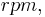$rpm,\,\!$ respectively. The analysis can be taken a step further to obtain an estimate of the reliability of the product at the use conditions using ReliaSoft's ALTA software. The data is entered into ALTA as shown next.

ALTA allows for modeling of the nature of relationship between life and stress. It is assumed that the relation between life of the product and temperature follows the Arrhenius relation while the relation between life and fan-speed follows the inverse power law relation.[ReliaSoft 2007a] Using these relations, ALTA fits the following model for the data: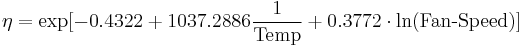$\eta =\exp [-0.4322+1037.2886\frac{1}{\text{Temp}}+0.3772\cdot \ln (\text{Fan-Speed})]\,\!$

Based on this model, the B10 life of the product at the use conditions is obtained as shown next. The Weibull reliability equation is: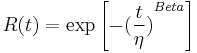$R(t)=\exp \left[ -{{(\frac{t}{\eta })}^{Beta}} \right]\,\!$

Substituting the value of$\eta \,\!$ from the ALTA model and the value of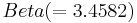$Beta(=3.4582)\,\!$ as obtained from ALTA, the reliability equation becomes: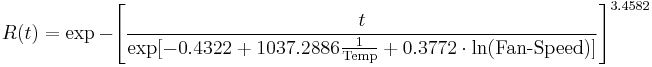$R(t)=\exp -{{\left[ \frac{t}{\exp [-0.4322+1037.2886\tfrac{1}{\text{Temp}}+0.3772\cdot \ln (\text{Fan-Speed})]} \right]}^{3.4582}}\,\!$

Finally, substituting the use conditions (Temp$=298\,\!$$K\,\!$, Fan-Speed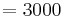$=3000\,\!$$rpm\,\!$) and the desired reliability value of 90%, the B10 life is obtained: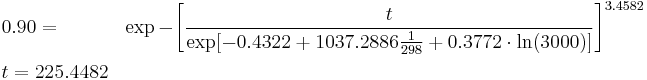\begin{align} & 0.90= & \exp -{{\left[ \frac{t}{\exp [-0.4322+1037.2886\tfrac{1}{298}+0.3772\cdot \ln (3000)]} \right]}^{3.4582}} \\ & t= 225.4482 \end{align}\,\!

Therefore, at the use conditions, the B10 life of the product is 225 hours. This result and other reliability metrics can be directly obtained from ALTA.